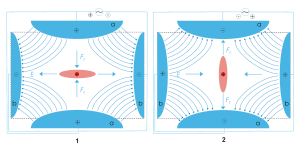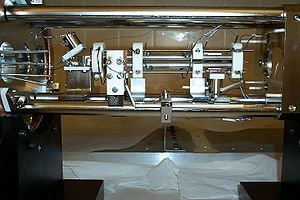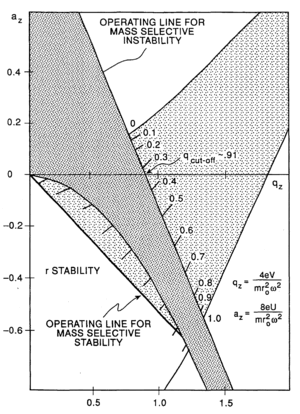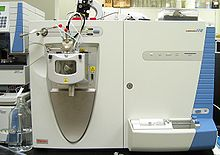Scheme of a Quadrupole ion trap of classical setup with a particle of positive charge (dark red), surrounded by a cloud of similarly charged particles (light red). The electric field E (blue) is generated by a quadrupole of endcaps (a, positive) and a ring electrode (b). Picture 1 and 2 show two states during an AC cycle.

A quadrupole ion trap exists in both linear and 3D (Paul Trap, QIT) varieties and refers to an ion trap that uses constant DC and radio frequency (RF) oscillating AC electric fields to trap ions. It is commonly used as a component of a mass spectrometer. The invention of the 3D quadrupole ion trap itself is attributed to Wolfgang Paul who shared the Nobel Prize in Physics in 1989 for this work.

## Theory

The 3D trap itself generally consists of two hyperbolic metal electrodes with their foci facing each other and a hyperbolic ring electrode halfway between the other two electrodes. The ions are trapped in the space between these three electrodes by AC (oscillating, non-static) and DC (non-oscillating, static) electric fields. The AC radio frequency voltage oscillates between the two hyperbolic metal end cap electrodes if ion excitation is desired; the driving AC voltage is applied to the ring electrode. The ions are first pulled up and down axially while being pushed in radially. The ions are then pulled out radially and pushed in axially (from the top and bottom). In this way the ions move in a complex motion that generally involves the cloud of ions being long and narrow and then short and wide, back and forth, oscillating between the two states. Since the mid-1980s most 3D traps (Paul traps) have used ~1 mtorr of helium. The use of damping gas and the mass-selective instability mode developed by Stafford et al. led to the first commercial 3D ion traps.Linear Ion Trap at the University of Calgary

The quadrupole ion trap has two configurations: the three dimensional form described above and the linear form made of 4 parallel electrodes. A simplified rectilinear configuration has also been used. The advantage of the linear design is in its simplicity, but this leaves a particular constraint on its modeling. To understand how this originates, it is helpful to visualize the linear form. The Paul trap is designed to create a saddle-shaped field to trap a charged ion, but with a quadrupole, this saddle-shaped electric field cannot be rotated about an ion in the centre. It can only 'flap' the field up and down. For this reason, the motions of a single ion in the trap are described by the Mathieu Equations. These equations can only be solved numerically, or equivalently by computer simulations.

The intuitive explanation and lowest order approximation is the same as strong focusing in accelerator physics. Since the field affects the acceleration, the position lags behind (to lowest order by half a period). So the particles are at defocused positions when the field is focusing and vice versa. Being farther from center, they experience a stronger field when the field is focusing than when it is defocusing.

### Equations of motion

Ions in a quadrupole field experience restoring forces that drive them back toward the center of the trap. The motion of the ions in the field is described by solutions to the Mathieu equation. When written for ion motion in a trap, the equation is$\frac{d^2u}{d\xi^2}+[a_u-2q_u\cos (2\xi) ]u=0 \qquad\qquad (1) \!$

where u represents the x, y and z coordinated, ξ is a dimensionless parameter given by$\xi=\Omega t/2\$, and$a_u\,$ and$q_u\,$ are dimensionless trapping parameters. The parameter$\Omega\,$ is the radial frequency of the potential applied to the ring electrode. By substituting the expression for ξ into Equation 1, it can be shown that$\frac{d^2u}{dt^2} = \frac{\Omega^2}{4} \frac{d^2u}{d\xi^2} \qquad\qquad (2) \!$

Substituting Equation 2 into the Matthieu Equation 1 yields$\frac{4}{\Omega^2}\frac{d^2u}{dt^2} + [a_u-2q_u\cos (\Omega t) ]u=0 \qquad\qquad (3) \!$.

Reorganizing terms shows us that$m \frac{d^2u}{dt^2} + m \frac{\Omega^2}{4}[a_u-2q_u\cos (\Omega t) ]u=0 \qquad\qquad (3) \!$.

By Newton's laws of motion, the above equation represents the force on the ion. This equation can be exactly solved using Floquet theorem or the standard techniques of multiple scale analysis . The particle dynamics and time averaged density of charged particles in a Paul trap can also be obtained by the concept of ponderomotive force.

The forces in each dimension are not coupled, thus the force acting on an ion in, for example, the x dimension is$F_x = ma = m\frac{d^2x}{dt^2} = -e \frac{\partial \phi}{\partial x} \qquad\qquad (4) \!$

Here,$\phi\,$ is the quadrupolar potential, given by$\phi=\frac{\phi_0}{r_0^2} \big( \lambda x^2 + \sigma y^2 + \gamma z^2 \big) \qquad\qquad (5) \!$

where$\phi _0\,$ is the applied electric potential and$\lambda\,$,$\sigma\,$, and$\gamma\,$ are weighting factors, and$r_0\,$ is a size parameter constant. In order to satisfy the Laplace Condition,$\nabla^2\phi_0=0\,$, it can be shown that$\lambda + \sigma + \gamma =0 \,$.

For an ion trap,$\lambda = \sigma =1 \,$ and$\gamma =-2 \,$ and for a quadrupole mass filter,$\lambda = -\sigma =1 \,$ and$\gamma =0 \,$.

Transforming Equation 5 into a cylindrical coordinate system with${x}={r} \,\cos\theta$,${y}={r} \, \sin\theta$, and${z}={z} \,$ and applying the pythagorean trigonometric identity$\sin^2 \theta + \cos^2 \theta = 1 \,$ givesDiagram of the stability regions of a quadrupole ion trap according to the voltage and frequency applied to the ion trap elements.$\phi_{r,z} = \frac{\phi_0}{r_0^2} \big( r^2 - 2z^2 \big) . \qquad\qquad (6) \!$

The applied electric potential is a combination of RF and DC given by$\phi_0 = U + V\cos \Omega t .\qquad\qquad (7) \!$

where Ω = 2πν and ν is the applied frequency in hertz.

Substituting Equation 7 into Equation 5 with λ = 1 gives$\frac{\partial \phi}{\partial x} = \frac {2x}{r_0^2} \big( U + V\cos \Omega t \big) . \qquad\qquad (8) \!$

Substituting Equation 8 into Equation 4 leads to$m\frac {d^2x}{dt^2} = - \frac {2e}{r_0^2} \big( U + V\cos \Omega t \big) x . \qquad\qquad (9) \!$

Comparing terms on the right hand side of Equation 1 and Equation 9 leads to$a_x = \frac {8eU} {m r_0^2 \Omega^2} \qquad\qquad (10) \!$

and$q_x = - \frac {4eV} {m r_0^2 \Omega^2} . \qquad\qquad (11) \!$

Further$q_x = q_y\,$,$a_z = -\frac {8eU} {m r_0^2 \Omega^2} \qquad\qquad (12) \!$

and$q_z = \frac {4eV} {m r_0^2 \Omega^2} . \qquad\qquad (13) \!$

The trapping of ions can be understood in terms of stability regions in qu and au space.

## Linear ion trapThe linear ion trap uses a set of quadrupole rods to confine ions radially and a static electrical potential on-end electrodes to confine the ions axially. The linear form of the trap can be used as a selective mass filter, or as an actual trap by creating a potential well for the ions along the axis of the electrodes. Advantages of the linear trap design are increased ion storage capacity, faster scan times, and simplicity of construction (although quadrupole rod alignment is critical, adding a quality control constraint to their production. This constraint is additionally present in the machining requirements of the 3D trap).

## Cylindrical ion trap

Cylindrical ion traps have a cylindrical rather than a hyperbolic ring electrode. This configuration has been used in miniature arrays of traps.

Wikimedia Foundation. 2010.

### Look at other dictionaries:

• Ion trap — ). An ion trap mass spectrometer may incorporate a Penning trap (Fourier transform ion cyclotron resonance) [cite journal | title = High accuracy mass spectrometry with stored ions | author = Blaum, Klaus | journal = Physics Reports | volume =… …   Wikipedia

• Quadrupole mass analyzer — The quadrupole mass analyzer is one type of mass analyzer used in mass spectrometry. As the name implies, it consists of 4 circular rods, set perfectly parallel to each other.cite book|last=de Hoffmann|first=Edmond|coauthors=Vincent… …   Wikipedia

• Quadrupole — A quadrupole or quadrapole is one of a sequence of configurations of for example electric charge or current, or gravitational mass that can exist in ideal form, but it is usually just part of a multipole expansion of a more complex structure… …   Wikipedia

• Triple quadrupole mass spectrometer — Waters Quattro II triple quadropole mass spectrometer (center). This photo was taken in the old mass spec facility in Whitmore Lab of Pennsylvania State University. A triple quadrupole mass spectrometer is a tandem mass spectrometer consisting of …   Wikipedia

• Fourier transform ion cyclotron resonance — Infobox chemical analysis name = Fourier transform ion cyclotron resonance caption =A FTMS instrument at the Pacific Northwest National Laboratory, USA acronym = FTMS, FTICR classification =Mass spectrometry analytes = manufacturers = related =… …   Wikipedia

• DART ion source — A capsule being analyzed is held in the sample chamber between the DART ion source (right) and the spectrometer inlet (cone on left). DART (Direct Analysis in Real Time) is an atmospheric pressure ion source that instantaneously ionizes gases,… …   Wikipedia

• Combined RF trap — A combined RF trap is a combination of a Quadrupole ion trap (QIT, also called a Paul trap) and a Penning trap. One of the main bottlenecks of a QIT is that it can confine only single charged species or multiple species with similar masses.… …   Wikipedia

• Penning trap — Penning traps are devices for the storage of charged particles using a constant static magnetic field and a spatially inhomogeneous static electric field. This kind of trap is particularly well suited to precision measurements of properties of… …   Wikipedia

• Trapped ion quantum computer — A Trapped ion quantum computer is a type of quantum computer. Ions, or charged atomic particles, can be confined and suspended in free space using electromagnetic fields. Qubits are stored in stable electronic states of each ion, and quantum… …   Wikipedia

• Mass spectrometry — (MS) is an analytical technique that measures the mass to charge ratio of charged particles. It is used for determining masses of particles, for determining the elemental composition of a sample or molecule, and for elucidating the chemical… …   Wikipedia